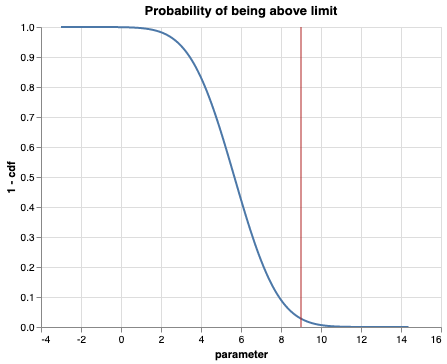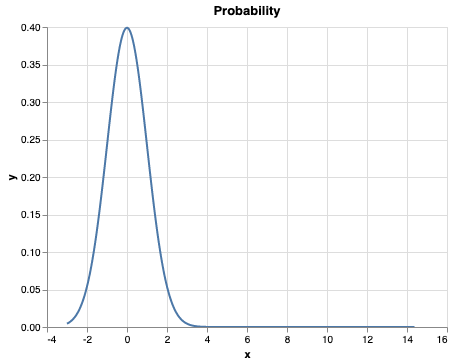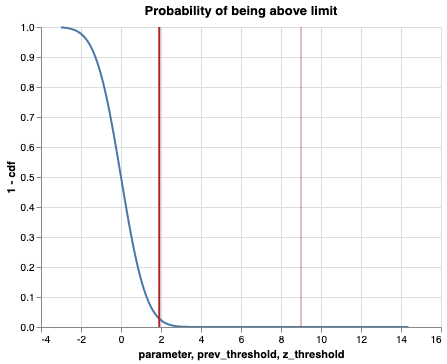# Calculating the Probability of some Event (E)

Probability can be used to describe the relationship between given values and the chance/probability of some event occuring. This post will outline how we describe probability of some event given a fitted distribution. We will fit a distribution to then calculate the survival function using the z-score of our limit given a fitted distribution.

from scipy import stats
import pandas as pd
import numpy as np
import altair as alt
alt.data_transformers.enable('json')
DataTransformerRegistry.enable('json')
df = pd.read_csv('example_parameter.csv')

# maximum likelihood estimate

In essence we either try distributions until it fits the data the best, or we know a analytical solution for the distribution at hand and get the best fitted distribution of the data right away.

mu, std = stats.norm.fit(df)
mu, std
(5.669365294764912, 1.7405441476684247)
# x between -3 and above with .001 steps.
x_axis = np.arange(
-3,
mu + 5 * std,
0.001
)

# Mean = mu, SD = std.
y_axis = stats.norm.pdf(
x_axis,
mu,
std
)

# plot
df = pd.DataFrame()
df['x'] = x_axis
df['y'] = y_axis
alt.Chart(data=df).mark_line().encode(
x='x:Q',
y='y:Q'
).properties(
title='Probability'
)since we have now fitted a curve around a mu (i.e. not around 0) which is the standard way of showing a distribution. We will here demonstrate how to calculate the likelihood of being above a certain value based on the data that we have seen using the survival function.

# 2. probability of the survival function

### is the where cdf is the cumulative distribution function

# plot
x_axis = x_axis

y_axis = 1 - stats.norm.cdf(x_axis, mu, std)

df = pd.DataFrame()
df['parameter'] = x_axis
df['1 - cdf'] = y_axis
line = alt.Chart(df).mark_line().encode(
x='parameter:Q',
y='1 - cdf:Q'
).properties(
title='Probability of being above limit'
)

vertical_y = np.arange(
0,
2,
0.001
)
df = pd.DataFrame()
df['vertical_y'] = vertical_y
df['limit'] = limit

threshold = pd.DataFrame([{"threshold": limit}])
rule = alt.Chart(threshold).mark_rule(color='firebrick').encode(
alt.X('threshold:Q', title='parameter')
)

line + rule# 3. take the z-score of the fitted distribution

Since each distribution is centered around we need to center our limit value against a normal distribution. This is where we calculate the z-score from the distribution.

which says how many standard deviations we are from its .

recall again that we want to see the probability of somethign accuring above the limit value. i.e. what is the probability that we exceed this value

# set the limit of the measure
limit = 9
z = (limit - mu) / std
z
1.9135594519085861

since we now have center our limit value around the we need to apply the calculation for the probability given a distrubution that we assume with this calculation.

meaning a and a

# x between -3 and above with .001 steps.
x_axis = np.arange(
-3,
mu + 5 * std,
0.001
)

# Mean = mu, SD = std.
y_axis = stats.norm.pdf(
x_axis,
0,
1
)

# plot
df = pd.DataFrame()
df['x'] = x_axis
df['y'] = y_axis
alt.Chart(data=df).mark_line().encode(
x='x:Q',
y='y:Q'
).properties(
title='Probability'
)# With z score we can now use a normal distribution for our calculations

# plot
x_axis = x_axis

y_axis = 1 - stats.norm.cdf(x_axis, 0, 1)

df = pd.DataFrame()
df['parameter'] = x_axis
df['1 - cdf'] = y_axis
line = alt.Chart(df).mark_line().encode(
x='parameter:Q',
y='1 - cdf:Q'
).properties(
title='Probability of being above limit'
)

vertical_y = np.arange(
0,
2,
0.001
)

threshold = pd.DataFrame([
{"prev_threshold": limit},
{"z_threshold": z}
])

rule = alt.Chart(threshold).mark_rule(color='firebrick').encode(
x='prev_threshold:Q',
opacity=alt.OpacityValue(0.6)
)

rule2 = alt.Chart(threshold).mark_rule(color='firebrick').encode(
alt.X('z_threshold:Q'),
size=alt.SizeValue(2)
)

line + (rule + rule2)With the calculated z-score, we now have a way of also using the normal distribution without the need to explicitly tell for the distribution.

## Back to the real world!!

Instead of writing the survival function which we will explicitly calculate the probability like so:

# score of the survival function
p = stats.norm.sf(limit, mu, std)
p
0.02783823512029548

We can now instead use the z-score for each call to a distribution.

# score of the survival function
p = stats.norm.sf(z)
p
0.02783823512029548

And we can see that it gives the same probability, just using different scales for the ,Hi, I'm Eric. I'm a software engineer and data scientist based in Lisbon. You can follow me on Twitter, see some of my work on GitHub,